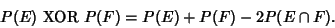## Exactly One

Exactly one'' means one and only one,'' sometimes also referred to as Just One.'' J. H. Conway has also humorously suggested onee'' (one and only one) by analogy with Iff (if and only if), twoo'' (two and only two), and threee'' (three and only three). This refinement is sometimes needed in formal mathematical discourse because, for example, if you have two apples, you also have one apple, but you do not have exactly one apple.

In 2-valued Logic, exactly one is equivalent to the exclusive or operator XOR,